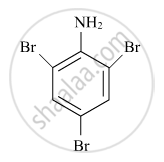# ISC (Arts) Class 12 - CISCE Important Questions for Chemistry (Theory)

Subjects
Topics
Subjects
Popular subjects
Topics
Chemistry (Theory)
< prev  1 to 20 of 487  next >

Define terms: Fuel cell

Appears in 14 question papers
Chapter: [6.02] Galvanic cells, mechanism of current production in a galvanic cell;
Concept: Batteries > Primary Batteries

Write the equations involved in the following reactions:

Reimer-Tiemann reaction

Appears in 10 question papers
Chapter: [12.03] Distinction between primary, secondary and tertiary alcohols
Concept: Chemical Properties of Phenol

Give reasons for the following:

Primary amines have higher boiling point than tertiary amines.

Appears in 6 question papers
Chapter: [0.15] Cyanides, Isocyanides, Nitro compounds, Amines and Diazonium Salt
Concept: Physical Properties of Amines

On the basis of crystal field theory, write the electronic configuration for d4 ion if ∆0 < P.

Appears in 5 question papers
Chapter: [0.07] Coordination Compounds
Concept: Bonding in Coordination Compounds > Crystal Field Theory (CFT)

State Henry’s law.

Appears in 5 question papers
Chapter: [1.01] Raoult's law and colligative properties
Concept: Solubility > Solubility of a Gas in a Liquid

Account for the following :

Zn is not considered as a transition element.

Appears in 4 question papers
Chapter: [0.1] Chemistry of Transition and Inner-Transition Elements: d-Block: 3d, 4d and 5d series f-Block: 4f and 5f series
Concept: General Properties of the Transition Elements (D-block)

Define azeotropes.

Appears in 4 question papers
Chapter: [1.01] Raoult's law and colligative properties
Concept: Vapour Pressure of Liquid > Vapour Pressure of Liquid- Liquid Solutions

Write the chemical equations to illustrate the following name reactions : Rosenmund reduction

Appears in 4 question papers
Chapter: [14.02] Acid derivatives
Concept: Aldehydes and Ketones > Preparation of Aldehydes

An element crystallizes in a f.c.c. lattice with a cell edge of 250 pm. Calculate the density if 300 g of this element contains 2 × 1024 atoms.

Appears in 3 question papers
Chapter: [0.02] States of Matters: Structure and Properties Solid State
Concept: Calculations Involving Unit Cell Dimensions

An element with density 2.8 g cm–3 forms a f.c.c. unit cell with edge length 4 x 10–8 cm. Calculate the molar mass of the element.

(Given : NA = 6.022 x 1023 mol –1)

Appears in 3 question papers
Chapter: [0.02] States of Matters: Structure and Properties Solid State
Concept: Calculations Involving Unit Cell Dimensions

Write the IUPAC name of the given compound:Appears in 3 question papers
Chapter: [0.07] Coordination Compounds
Concept: Nomenclature of Coordination Compounds - Naming of Mononuclear Coordination Compounds

When a co-ordination compound CrCl3.6H2O is mixed with AgNO3, 2 moles of AgCl are precipitated per mole of the compound. Write

(i) Structural formula of the complex.

(ii) IUPAC name of the complex.

Appears in 3 question papers
Chapter: [0.07] Coordination Compounds
Concept: Nomenclature of Coordination Compounds - Naming of Mononuclear Coordination Compounds

Write down the IUPAC name of the following complex: [Cr(NH3)2Cl2(en)]Cl (en = ethylenediamine)

Appears in 3 question papers
Chapter: [0.07] Coordination Compounds
Concept: Nomenclature of Coordination Compounds - Naming of Mononuclear Coordination Compounds

Draw the geometrical isomers of complex [Pt(NH3)2Cl2].

Appears in 3 question papers
Chapter: [0.07] Coordination Compounds
Concept: Isomerism in Coordination Compounds > Stereoisomerism

Write the IUPAC name of the complex [Cr(NH3)4 Cl2]Cl.

Appears in 3 question papers
Chapter: [0.07] Coordination Compounds
Concept: Nomenclature of Coordination Compounds - Naming of Mononuclear Coordination Compounds

Using IUPAC norms write the formulae for Potassium trioxalatoaluminate(III)

Appears in 3 question papers
Chapter: [0.07] Coordination Compounds
Concept: Importance and Applications of Coordination Compounds

What is meant by positive deviations from Raoult's law? Give an example. What is the sign of ∆mixH for positive deviation?

Appears in 3 question papers
Chapter: [1.01] Raoult's law and colligative properties
Concept: Vapour Pressure of Liquid > Vapour Pressure of Liquid- Liquid Solutions

The rate constant for the first-order decomposition of H2O2 is given by the following equation:

logk=14.2-(1.0xx10^4)/TK

Calculate Ea for this reaction and rate constant k if its half-life period be 200 minutes.

(Given: R = 8.314 JK–1 mol–1)

Appears in 3 question papers
Chapter: [3.05] The concept of energy
Concept: Temperature Dependence of the Rate of a Reaction

What is rate of reaction?

Appears in 3 question papers
Chapter: [3.08] Rate of Reaction
Concept: Rate of a Chemical Reaction

From the given cells:

Lead storage cell, Mercury cell, Fuel cell and Dry cell

(i) Which cell is used in hearing aids?

(ii) Which cell was used in Apollo Space Programme?

(iii) Which cell is used in automobiles and inverters?

(iv) Which cell does not have long life?

Appears in 3 question papers
Chapter: [6.02] Galvanic cells, mechanism of current production in a galvanic cell;
Concept: Batteries > Primary Batteries
< prev  1 to 20 of 487  next >